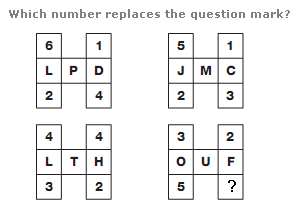# Puzzles - Logical puzzles

### Exercise :: Logical puzzlesAnswer : Alarm clock Explanation : The Alarm clock = 7, the Adaptor = 5 and the Bulb = 2

View Answer & ExplanationAnswer : A Explanation : Working from left to right, top row then bottom row, the first grid contains a sequence of 2 black dots and a sequence of 3. The next grid contains one of 3 and one of 4. Continue, adding 1 to each sequence every time.

View Answer & ExplanationAnswer : 3 Explanation : In each diagram, the numerical value of the left hand letter equals the product of the upper and lower left hand numbers, and the right hand letter equals the product of the upper and lower right hand numbers. The numerical value of the central letter equals the sum of the numerical values of the left and right hand letters.

View Answer & Explanation

#### Current Affairs 2019

Interview Questions and Answers